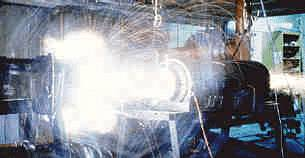# Rail GunA railgun uses the flow of electricity to accelerate a projectile to incredibly high velocities. It is distinct from other guns (or more correctly, cannons) by the fact that no chemical combustion is necessary. In fact, no gasses are used to accelerate the projectile. All the acceleration is done by the flow of electricity. Railguns are a relatively new technology and are much less efficient than other chemical means. Railguns that operate with a total efficiency of over two percent are considered highly efficient. The advantage of railguns is that they can accelerate a projectile much faster than is possible using any other means. To date, railguns can easily fire a projectile at over velocities of 10 kilometers per second. Velocities of approximately 150 kilometers per second are considered possible. By comparison, the maximum velocity that a light gas gun has fired a projectile is less than eight kilometers per second. HOW THEY WORK A railgun is essentially a linear DC motor. Instead of the current spinning an armature, the current pushes a projectile (see Figure below). A railgun consists of two parallel field-producing metal plates, the distance between them being the diameter of the projectile. A high-current DC source of electricity is discharged through the projectile via the two plates. This creates a force known as the Lorentz force that is perpendicular to the flow of the current. This propels the projectile in the opposite direction that the current came from.

Submitted by: Anthony McDowell